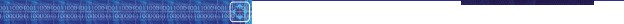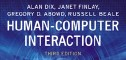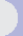HCI book > exercises > 17. system models > ex. 17.1 text onlyexercises17. models of the systemEXERCISE 17.1

Using the model-oriented approach with the example graphics program described in Section 17.2.3, specify the move operation as a schema which acts on the currently selected objects. Is the operation you have defined cumulative (two successive moves can be done as one move which is the sum of the other two) or is it 'forgetful?' Discuss the implications of the framing problem in your definition.

Students may not have a great familiarity with the Z notation used in this textbook, especially if they do not have a computer science background which emphasizes formal specification. Nevertheless, we will provide a model answer to this exercise in the Z notation and leave it to the instructor to decide whether an alternative language can be used to express the same ideas.

To accommodate the specification of the move operation, we will first need a function which allows us to add two coordinate points together. We just provide the declaration of this function here.

```|  _ + _: PointPointPoint
```

The motivation for this function is so that we can define a move operation with a single argument, called delta? below, which will specify how far to move the centres of every selected shape. This approach, using relative movements, will allow for two consecutive moves to be defined with the overall movement being the sum of the individual moves. Another approach (which would be quite cumbersome to define for a set of selected objects) would be to specify the move operation as an absolute operation, the argument(s) to the move explicitly indicating the new value for the centre(s) of each selected shape. This operation definition would not be cumulative.

Initially, we might want to define the move operation simply to update the centre position of all selected shapes.

```___Move___________________________________________________________
|  State
|  State'
|  delta? : Point
|____________________
|id : selectedshapes'(id).centre = shapes(id).centre + delta?
|________________________________________________________________________
```

There are a number of details in this defined operation which are left (dangerously) open to interpretation. Many of these are framing conditions on the Move operation. For example, what happens to shapes which are not selected? The normal intuition on this operation would be that they stay put, but the above definition actually allows them to change in any way - including the possibility of disappearing altogether. To ensure that unselected objects stay the same as they were before the operation, we would have to add a predicate to the definition.

```___Move___________________________________________________________
|  State
|  State'
|  delta? : Point
|____________________
|id : selectedshapes'(id).centre = shapes(id).centre + delta?
|id : (dom shapes - selected)shapes'(id) = shapes(id)
|________________________________________________________________________
```

We also need to explicitly state that no new shapes are introduced into the system, i.e., that the domain of shapes remains the same.

```___Move___________________________________________________________
|  State
|  State'
|  delta? : Point
|____________________
|id : selectedshapes'(id).centre = shapes(id).centre + delta?
|id : (dom shapes - selected)shapes'(id) = shapes(id).centre
|  dom shapes' = dom shapes
|________________________________________________________________________
```

For those familiar with Z, it is possible to collapse the last three predicates in the above schema to one predicate using functional overriding from the basic Z mathematical toolkit (see Spivey ).

Another framing issue which has impact on the interactive style of this graphics system is specifying what happens to the selected set of shapes after the move operation. Our current definition says nothing about this. Two clear options are whether the set of selected shapes remains the same or becomes the empty set (no selected objects). For reasons of compositionality (for defining consecutive move operations or consecutive operations targeted at the same set of shapes) we choose the former option, and specify this below.

```___Move___________________________________________________________
|  State
|  State'
|  delta? : Point
|____________________
|id : selectedshapes'(id).centre = shapes(id).centre + delta?
|id : (dom shapes - selected)shapes'(id) = shapes(id)
|  dom shapes' = dom shapes
|  selected' = selected
|________________________________________________________________________
```

[Note: Experienced Z users would probably choose the functional overriding operator () to more concisely express the first three predicates above.]

 Other exercises in this chapter ex.17.1 (ans), ex.17.2 (ans), ex.17.3 (ans), ex.17.4 (ans), ex.17.5 (tut), ex.17.6 (tut), ex.17.7 (tut), ex.17.8 (tut), ex.17.9 (tut)all exercises for this chapter